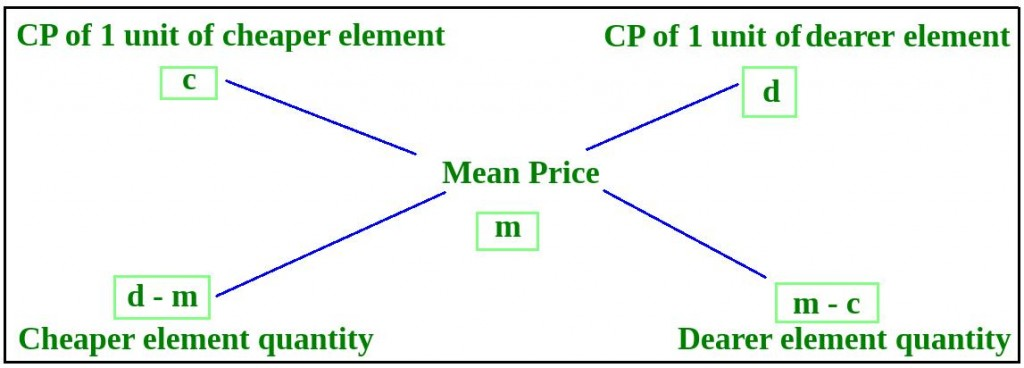Related Articles
Program to solve the Alligation Problem
• Last Updated : 21 Jan, 2019

Write a program to find the ratio in which a shopkeeper will mix two types of rice worth Rs.kg and Rs.kg, so that the average cost of the mixture is Rs.kg.

Examples:

```Input : X = 50, Y = 70, Z = 65
Output : Ratio = 1:3

Input : X = 1000, Y = 2000, Z = 1400
Output : Ratio = 3:2
```

## Recommended: Please try your approach on {IDE} first, before moving on to the solution.

According to Alligation rule, the ratio of the weights of two items mixed will be inversely proportional to the deviation of attributes of these two items from the average attribute of the resultant mixture.

```w1 / w2 = (d - m) / (m - c)
```Below program illustrate the above approach:

## C++

 `#include ``using` `namespace` `std;`` ` `// Function to find the ratio of two mixtures``void` `alligation(``float` `x, ``float` `y, ``float` `m)``{``    ``// Find the cheaper among x and y``    ``float` `c = (x <= y) ? x : y;``    ``// Find the dearer among x and y``    ``float` `d = (x >= y) ? x : y;`` ` `    ``// Find ratio r1:r2``    ``int` `r1 = d - m;``    ``int` `r2 = m - c;`` ` `    ``// Convert the ration into simpler form``    ``int` `gcd = __gcd(r1, r2);`` ` `    ``cout << r1 / gcd << ``":"` `<< r2 / gcd;``}`` ` `// Driver code``int` `main()``{``    ``float` `x, y, z;``    ``x = 50;``    ``y = 70;``    ``z = 65;`` ` `    ``alligation(x, y, z);`` ` `    ``return` `0;``}`

## Java

 `// Java implementation of the ``// above approach.``import` `java.util.*;`` ` `class` `solution``{`` ` `static` `float` `__gcd(``float` `a, ``float` `b)``{``    ``float` `dividend,divisor;``     ` `    ``// a is greater or equal to b``    ``if``(a>=b)``    ``dividend = a;``    ``else``    ``dividend = b;``     ` `    ``// b is greater or equal to a``    ``if``(a<=b)``    ``divisor = a;``    ``else``    ``divisor = b;``     ` `while``(divisor>``0``)``{``float` `remainder = dividend % divisor;``dividend = divisor;``divisor = remainder;`` ` `}``return` `dividend;``}``// Function to find the ratio of two mixtures``static` `void` `alligation(``float` `x, ``float` `y, ``float` `m)``{``    ``// Find the cheaper among x and y``    ``float` `c;``    ``if` `(x <= y)``    ``c = x;``    ``else``    ``c = y;``    ``// Find the dearer among x and y``    ``float` `d ;``    ``if` `(x >= y)``    ``d = x;``    ``else``    ``d = y;`` ` `    ``// Find ratio r1:r2``    ``float` `r1 = d - m;``    ``float` `r2 = m - c;`` ` `    ``// Convert the ration into simpler form``    ``float` `gcd = __gcd(r1, r2);`` ` `    ``System.out.println((``int``)(r1 / gcd)+``":"``+(``int``)(r2 / gcd));``}`` ` `// Driver code``public` `static` `void` `main(String args[])``{``    ``float` `x, y, z;``    ``x = ``50``;``    ``y = ``70``;``    ``z = ``65``;`` ` `    ``alligation(x, y, z);``}``}`` ` `// This code is contribuuted by``// Shashank_sharma`

## Python3

 `# Python 3 implementation of the ``# above approach.``from` `math ``import` `gcd`` ` `# Function to find the ratio ``# of two mixtures``def` `alligation(x, y, m):``     ` `    ``# Find the cheaper among x and y``    ``if` `(x <``=` `y):``        ``c ``=` `x``    ``else``:``        ``c ``=` `y``         ` `    ``# Find the dearer among x and y``    ``if` `(x >``=` `y):``        ``d ``=` `x``    ``else``:``        ``d ``=` `y`` ` `    ``# Find ratio r1:r2``    ``r1 ``=` `d ``-` `m``    ``r2 ``=` `m ``-` `c`` ` `    ``# Convert the ration into simpler form``    ``__gcd ``=` `gcd(r1, r2)`` ` `    ``print``(r1 ``/``/` `__gcd, ``":"``, r2 ``/``/` `__gcd)`` ` `# Driver code``if` `__name__ ``=``=` `'__main__'``:``    ``x ``=` `50``    ``y ``=` `70``    ``z ``=` `65`` ` `    ``alligation(x, y, z)`` ` `# This code is contributed by``# Surendra_Gangwar`

## C#

 `// C# implementation of the ``// above approach.``using` `System;`` ` `class` `GFG``{``    ``// Recursive function to return ``    ``// gcd of a and b ``    ``static` `int` `__gcd(``int` `a, ``int` `b) ``    ``{ ``        ``// Everything divides 0 ``        ``if` `(a == 0) ``            ``return` `b; ``        ``if` `(b == 0) ``            ``return` `a; ``         ` `        ``// base case ``        ``if` `(a == b) ``            ``return` `a; ``         ` `        ``// a is greater ``        ``if` `(a > b) ``            ``return` `__gcd(a - b, b); ``        ``return` `__gcd(a, b - a); ``    ``} ``     ` `    ``// Function to find the ratio of ``    ``// two mixtures``    ``static` `void` `alligation(``float` `x, ``                           ``float` `y, ``float` `m)``    ``{``        ``// Find the cheaper among x and y``        ``float` `c = (x <= y) ? x : y;``         ` `        ``// Find the dearer among x and y``        ``float` `d = (x >= y) ? x : y;``     ` `        ``// Find ratio r1:r2``        ``int` `r1 = (``int``)(d - m);``        ``int` `r2 = (``int``)(m - c);``     ` `        ``// Convert the ration into ``        ``// simpler form``        ``int` `gcd = __gcd(r1, r2);``     ` `        ``Console.Write(r1 / gcd + ``":"` `+ ``                      ``r2 / gcd);``    ``}``     ` `    ``// Driver code``    ``public` `static` `void` `Main()``    ``{``        ``float` `x, y, z;``        ``x = 50;``        ``y = 70;``        ``z = 65;``     ` `        ``alligation(x, y, z);``    ``}``}`` ` `// This code is contributed ``// by Akanksha Rai`

## PHP

 `= ``\$b``)``        ``\$dividend` `= ``\$a``;``    ``else``        ``\$dividend` `= ``\$b``;``     ` `    ``// b is greater or equal to a``    ``if``(``\$a` `<= ``\$b``)``        ``\$divisor` `= ``\$a``;``    ``else``        ``\$divisor` `= ``\$b``;``     ` `    ``while``(``\$divisor` `> 0)``    ``{``        ``\$remainder` `= ``\$dividend` `% ``\$divisor``;``        ``\$dividend` `= ``\$divisor``;``        ``\$divisor` `= ``\$remainder``;``    ``}``    ``return` `\$dividend``;``}`` ` `// Function to find the ratio of ``// two mixtures``function` `alligation(``\$x``, ``\$y``, ``\$m``)``{``    ``// Find the cheaper among x and y``    ``if` `(``\$x` `<= ``\$y``)``        ``\$c` `= ``\$x``;``    ``else``        ``\$c` `= ``\$y``;``     ` `    ``// Find the dearer among x and y``    ``if` `(``\$x` `>= ``\$y``)``        ``\$d` `= ``\$x``;``    ``else``        ``\$d` `= ``\$y``;`` ` `    ``// Find ratio r1:r2``    ``\$r1` `= ``\$d` `- ``\$m``;``    ``\$r2` `= ``\$m` `- ``\$c``;`` ` `    ``// Convert the ration into``    ``// simpler form``    ``\$gcd` `= __gcd(``\$r1``, ``\$r2``);`` ` `    ``echo` `(int)(``\$r1` `/ ``\$gcd``) . ``":"` `. ``         ``(int)(``\$r2` `/ ``\$gcd``);``}`` ` `// Driver code``\$x` `= 50;``\$y` `= 70;``\$z` `= 65;`` ` `alligation(``\$x``, ``\$y``, ``\$z``);`` ` `// This code is contributed by``// Mukul Singh``?>`
Output:
```1:3
```

Attention reader! Don’t stop learning now. Get hold of all the important DSA concepts with the DSA Self Paced Course at a student-friendly price and become industry ready.  To complete your preparation from learning a language to DS Algo and many more,  please refer Complete Interview Preparation Course.

My Personal Notes arrow_drop_up